## Example Questions

### Example Question #1 : How To Find The Solution To A Binomial Problem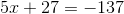Solve for.

Possible Answers: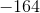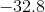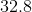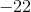Correct answer:Explanation:

Even though there are three terms in the equation, there are technically only two because it's a matter of collecting like terms.

The main objective in this problem is to solve for x. In order to do so, we need to get x by itself. The first step to accomplish this is to subtract 27 from the left side of the equation and do the same to the right. This follows the idea of "what you do to one side of an equation, you must do to the other." From here, you can collect like terms between -137 and -27 because they're both constants.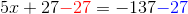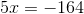The goal of getting x by itself on one side has been almost achieved. We still have that coefficient "5" that's being multiplied by x. In order to make 5x just x, 5x needs to be divided by 5 and therefore so does -164. Again, this follows the concept of mimicking actions on both sides of an equation.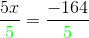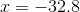### Example Question #1 : How To Find The Solution To A Binomial Problem

Choose the answer below that is one of the solutions to the following equation: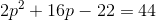Possible Answers: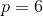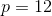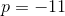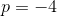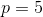Correct answer:Explanation:

The equation presented in the problem is:First, you need to get everything on one side of the equation: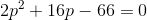Then, you can reduce by dividing everything by: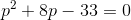Then, you factor: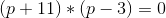Therefore,or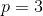.

### Example Question #3 : How To Find The Solution To A Binomial Problem

Choose the answer that is a potential solution to the binomial equation below: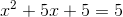Possible Answers: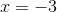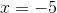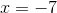Correct answer:Explanation:

The equation presented in the problem is:To solve, first get all terms on the same side of the equation: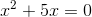Now, you've eliminated your third term, which makes factoring difficult. If it makes things easier, you can throw a zero back in the equation: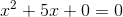Factor: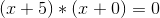Therefore:or### Example Question #4 : How To Find The Solution To A Binomial Problem

Choose the answer below that is a solution to the following binomial equation:Possible Answers: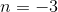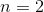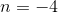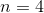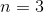Correct answer:Explanation:

First, get everything on one side of the equation: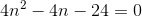Then, to make things easier, you can reduce by dividing everything by: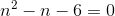Next, factor: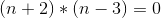Therefore: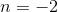or### Example Question #5 : How To Find The Solution To A Binomial Problem

Choose the answer which is a solution to the following binomial equation: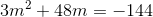Possible Answers: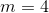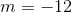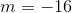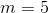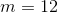Correct answer:Explanation:

To solve, first pull everything over to one side of the equation: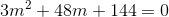Then, reduce by dividing by the greatest common denominator—in this case,: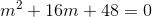Now, factor: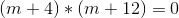Therefore: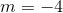or### Example Question #6 : How To Find The Solution To A Binomial Problem

Choose the answer below that is a possible solution to the following binomial equation: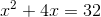Possible Answers: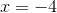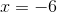Correct answer:Explanation:

The equation presented in the problem is:To solve this type of equation, you need to factor. First, get all of the terms of the equation on one side: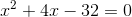Then, you need to find two factors that will give you the equation in its current form: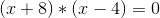Therefore,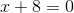and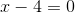, so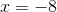or.is listed as an answer, and must therefore be correct.

### All ACT Math Resources